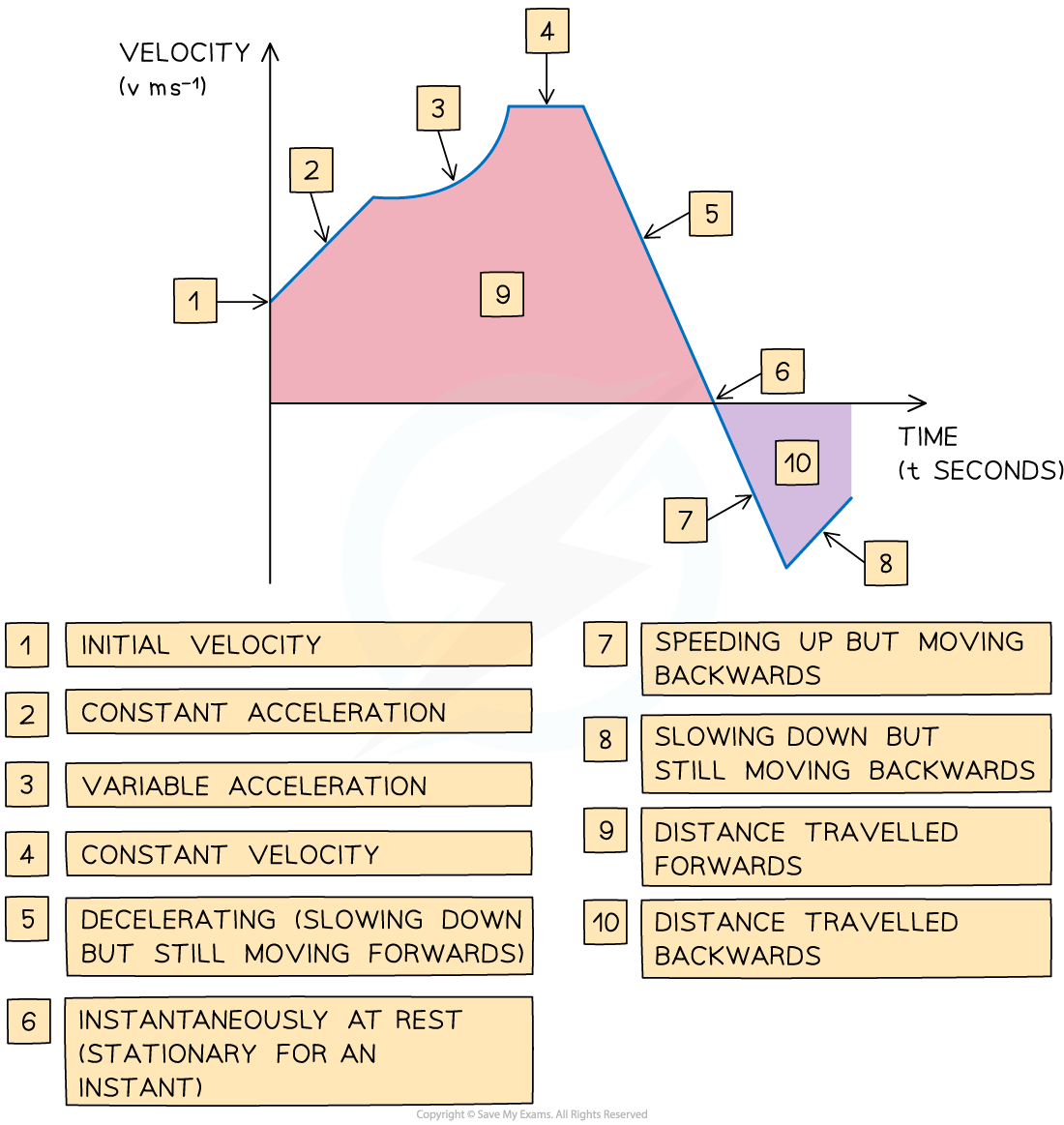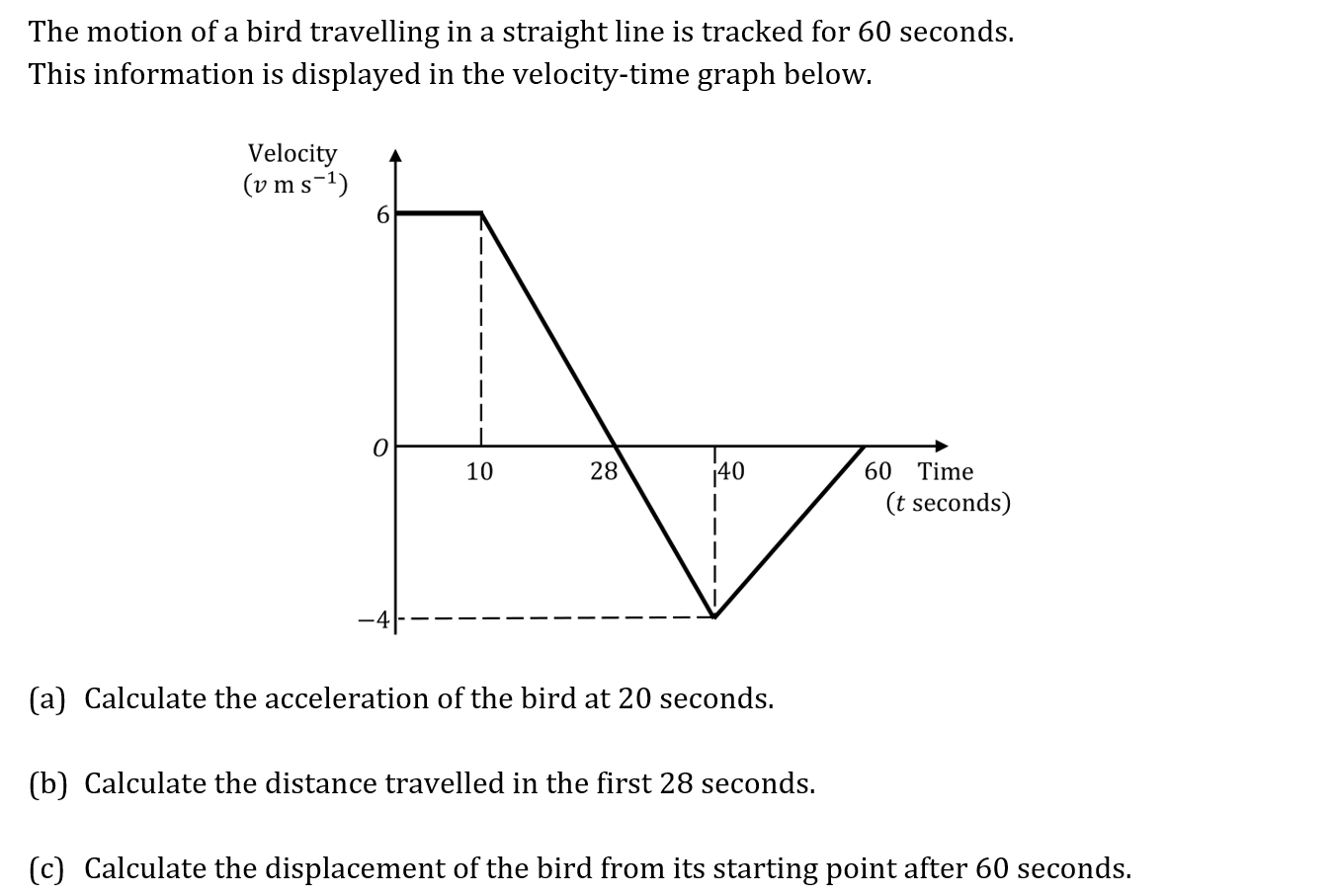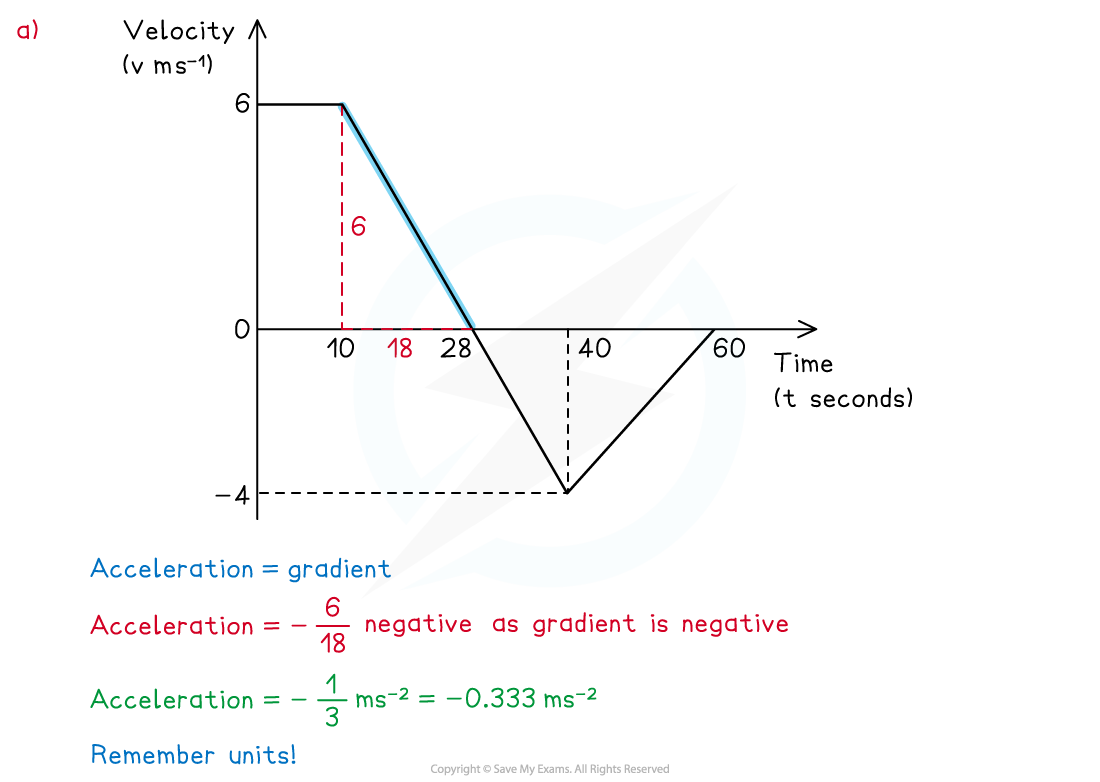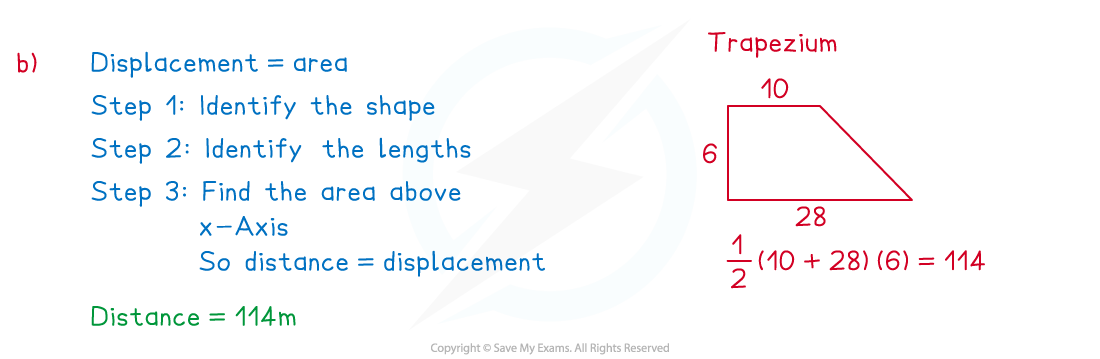# CIE A Level Maths: Mechanics复习笔记2.1.2 Velocity-Time Graphs

### Velocity-Time Graphs

#### What is a velocity-time graph?

• Velocity- time graphs show the velocity of an object as it moves in a straight line
• They show velocity (on the vertical axis) against time (on the horizontal axis)
• Velocity-time graphs can go below the horizontal axis whereas speed-time graphs can not

#### What are the key features of a velocity-time graph?

• The gradient of the graph equals the acceleration  of an object
• straight line shows that the object is accelerating at a constant rate
• horizontal line shows that the object is moving at a constant velocity
• The area between graph and the x-axis tells us the change in displacement of the object
• Graph above the x-axis means the object is moving forwards
• Graph below the x-axis means the object is moving backwards
• The total displacement of the object from its starting point is the sum of the areas above the x-axis minus the sum of the areas below the x-axis
• The total distance travelled by the object is the sum of all the areas
• If the graph touches the x-axis then the object is stationary at that time
• If the graph is above the x-axis then the object has positive velocity and is travelling forwards
• If the graph is below the x-axis then the object has negative velocity and is travelling backwards#### Worked Example(a) Calculate the acceleration of the bird at 20 seconds.(b) Calculate the distance travelled in the first 28 seconds.(c) Calculate the displacement of the bird from its starting point after 60 seconds.#### Exam Tip

• Be careful to spot if you are working with a distance-time graph or a velocity-time graph.
• Be careful to spot if you are working with a speed-time graph or a velocity-time graph.
• Check where the graph starts from on the y-axis, the velocity does not have to start at 0. For example, the scenario could be a car driving at a constant speed and the driver sees a hazard.
• Be extra vigilant when working with negative gradients or with graphs under the x-axis, it is easy to make mistakes with these.
• Speed is a scalar so it can not be negative whereas velocity can be.
• Make sure your units are consistent.